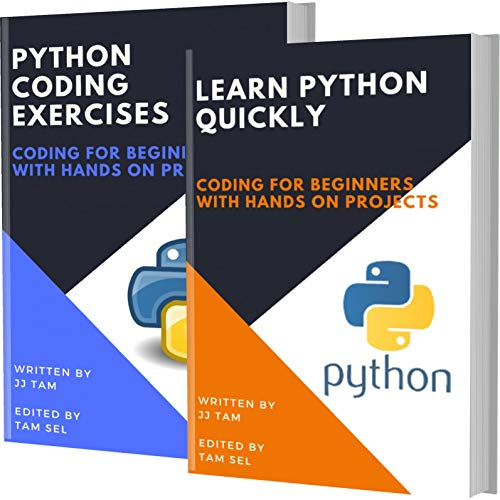Learn Python Quickly and Python Coding Exercises: Coding For Beginners - THE PIRATE BOOK

Friday, May 28, 2021Learn Python Quickly and Python Coding Exercises: Coding For Beginners

by
• Length: 128 pages
• Edition: 1
• Publication Date: 2020-12-27
• ISBN-10: B08RDY478F
Description

This book contains PYTHON BASICS AND PYTHON coding exercises for beginners. It’s useful Job interviews and college examinations.
This book only for absolute beginners.

LEARN PYTHON QUICKLY
Install python on MAC OS
Python: Hello World program
Python interactive command line
Python: Operators
Arithmetic Operators in python
Relational Operators in python
Assignment operators in python
Logical Operators in python
Bitwise Operators in python
Membership operators in python
Identity operators in python
Python: Short circuit operators
Strings in python
Python: if condition
Python: while statement
Python: for statement
Python: break statement
Python: continue statement
Python: functions
Python: functions: return statement
Python lists
Python: tuples
Python: Sets
Python modules
Python command line arguments
Python: File handling
Python: classes and objects
Python: Class Vs Instance variables
Python: Inheritance
Python: Exceptions
Python: Handling Exceptions
Python global keyword
Python: Get type of variable
Python Basic – Exercises
Get Python version
Display current date and time
Print the calendar
Computes the value of n+nn+nnn
Calculate number of days
volume of a sphere in Python
Compute the area of Triangle
Compute the GCD
Calculate The LCM
Convert feet and inches to centimeters
Convert time – seconds
Convert seconds to day
Calculate BMS
Sort three integers
Get system time
Check a number
Python code to Remove first item
Filter positive numbers
Count the number 4
Find a number even or odd
Get n copies of a given string
Print out a list which are not present in other list
Display details name, age, address in three different lines
Program to solve
Future value of amount
Check whether a file exists
Convert the distance
Sum all the items
Multiplies all the items
Get the largest number
Get the smallest number
Remove duplicates
Clone or copy a list
Difference between the two lists
Generate all permutations
Find the second smallest
Get unique values
Get the frequency of the elements
Generate all sublists
Find common items
Create a list
Remove consecutive duplicates
Flatten a nested